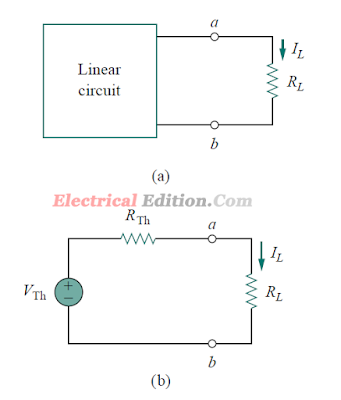# Thevenin’s Theorem - Dependent & Independent Sources

### Thevenin’s Theorem:-

Thevenin’s theorem states that a linear two-terminal circuit can be replaced by an equivalent circuit consisting of a voltage source VTh in series with a resistor RTh, where VTh is the open-circuit voltage at the terminals and RTh is the input or equivalent resistance at the terminals when the independent sources are turned off.

It often occurs in practice that a particular element in a circuit is variable (usually called the load) while other elements are fixed. As a typical example, a household outlet terminal may be connected to different appliances constituting a variable load. Each time the variable element is changed, the entire circuit has to be analyzed all over again. To avoid this problem, Thevenin’s theorem provides a technique by which the fixed part of the circuit is replaced by an equivalent circuit. According to Thevenin’s theorem, the linear circuit in Fig.(a) can be replaced by that in Fig. (b). (The load in Fig. may be a single resistor or another circuit.) The circuit to the left of the terminals a-b in Fig.(b) is known as the Thevenin equivalent circuit; it was developed in 1883 by M. Leon Thevenin (1857 1926), a French telegraph engineer.First Image
Our major concern right now is how to find the Thevenin equivalent voltage VTh and resistance RTh. To do so, suppose the two circuits in Fig. are equivalent. Two circuits are said to be equivalent if they have the same voltage-current relation at their terminals. Let us find out what will make the two circuits in above Fig. equivalent. If the terminals a-b are made open-circuited (by removing the load), no current flows, so that the open-circuit voltage across the terminals a-b in above Fig.(a) must be equal to the voltage source VTh in above Fig.(b), since the two circuits are equivalent. Thus VTh is the open-circuit voltage across the terminals as shown in below Fig.(a); that is, VTh = VocSecond image

Again, with the load disconnected and terminals a-b open-circuited, we turn off all independent sources. The input resistance (or equivalent resistance) of the dead circuit at the terminals a-b in Fig.first image(a) must be equal to RTh in Fig.first image(b) because the two circuits are equivalent. Thus, RTh is the input resistance at the terminals when the independent sources are turned off, as shown in Fig.first image(b); that is,

RTh = Rin

To apply this idea in finding the Thevenin resistance RTh, we need to consider two cases.

### Thevenin’s Theorem -With Independent Sources:-

CASE 1 If the network has no dependent sources, we turn off all independent
sources. RTh is the input resistance of the network looking
between terminals a and b, as shown in Fig. second image(b).

### Thevenin’s Theorem - Dependent & Independent Sources:-

CASE 2 If the network has dependent sources, we turn off all independent sources. As with superposition, dependent sources are not to be turned off because they are controlled by circuit variables. We apply a voltage source vo at terminals a and b and determine the resulting current io. Then RTh = vo/io, as shown in Fig. third image(a). Alternatively, we may insert a current source io at terminals a-b as shown in Fig.third image(b) and find the terminal voltage vo. Again RTh = vo/io. Either of the two approaches will give the same result. In either approach we may assume any value of vo and io. For example, we may use vo = 1 V or io = 1 A, or even use unspecified values of vo or io.

It often occurs that RTh takes a negative value. In this case, the negative resistance (v = −iR) implies that the circuit is supplying power.Third Image

This is possible in a circuit with dependent sources; Example  will illustrate this.Thevenin’s theorem is very important in circuit analysis. It helps simplify a circuit. A large circuit may be replaced by a single independent voltage source and a single resistor. This replacement technique is a powerful tool in circuit design.Fourth image

As mentioned earlier, a linear circuit with a variable load can be replaced by the Thevenin equivalent, exclusive of the load. The equivalent network behaves the same way externally as the original circuit. Consider a linear circuit terminated by a load RL, as shown in Fig.fourth image(a).The current IL through the load and the voltage VL across the load are easily determined once the Thevenin equivalent of the circuit at the load’s terminals is obtained, as shown in Fig.fourth image(b). From Fig.fourth image(b), we obtain

Note: from Fig. fourth image(b) that the Thevenin equivalent is a simple voltage divider, yielding VL by mere inspection